# Matplotlib 基础¶

Matplotlib是一个类似Matlab的工具包，主页地址为

http://matplotlib.org

In :
%pylab

Using matplotlib backend: Qt4Agg
Populating the interactive namespace from numpy and matplotlib


## plot 二维图¶

plot(y)
plot(x, y)
plot(x, y, format_string)


In :
%matplotlib inline
x = linspace(0, 2 * pi, 50)
plot(sin(x))

Out:
[<matplotlib.lines.Line2D at 0xa086fd0>]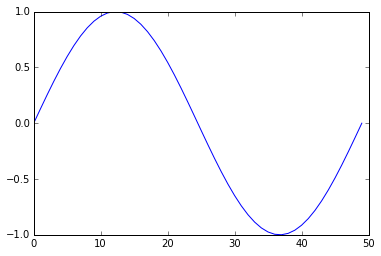In :
plot(x, sin(x))

Out:
[<matplotlib.lines.Line2D at 0xa241898>]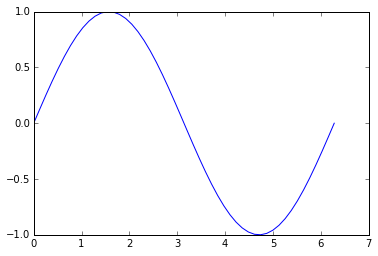In :
plot(x, sin(x),
x, sin(2 * x))

Out:
[<matplotlib.lines.Line2D at 0xa508b00>,
<matplotlib.lines.Line2D at 0xa508d30>]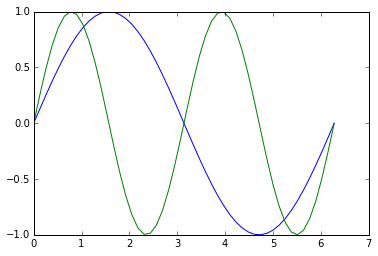In :
plot(x, sin(x), 'r-^')

Out:
[<matplotlib.lines.Line2D at 0xba6ea20>]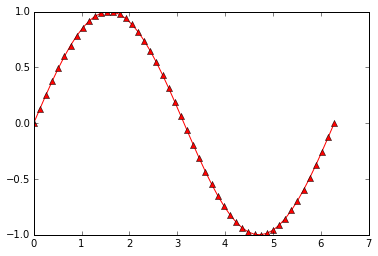In :
plot(x, sin(x), 'b-o',
x, sin(2 * x), 'r-^')

Out:
[<matplotlib.lines.Line2D at 0xbcf1710>,
<matplotlib.lines.Line2D at 0xbcf1940>]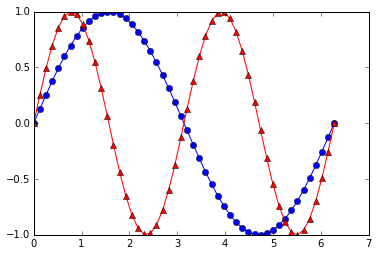## scatter 散点图¶

scatter(x, y)
scatter(x, y, size)
scatter(x, y, size, color)


In :
plot(x, sin(x), 'bo')

Out:
[<matplotlib.lines.Line2D at 0xbd6c0b8>]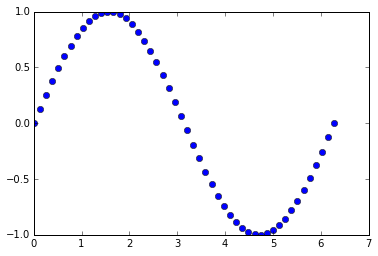In :
scatter(x, sin(x))

Out:
<matplotlib.collections.PathCollection at 0xbd996d8>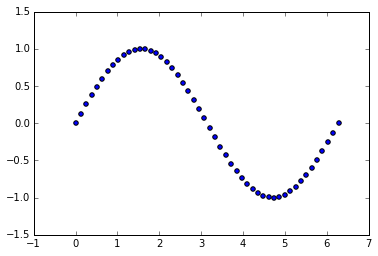In :
x = rand(200)
y = rand(200)
size = rand(200) * 30
color = rand(200)
scatter(x, y, size, color)
# 显示颜色条
colorbar()

Out:
<matplotlib.colorbar.Colorbar instance at 0x000000000C31F448>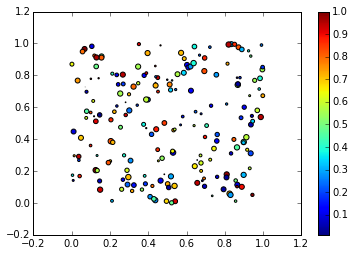## 多图¶

In :
t = linspace(0, 2*pi, 50)
x = sin(t)
y = cos(t)
figure()
plot(x)
figure()
plot(y)

Out:
[<matplotlib.lines.Line2D at 0xc680cf8>]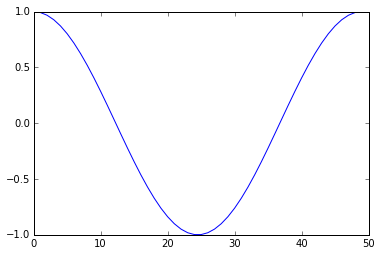subplot(row, column, index)
In :
subplot(1, 2, 1)
plot(x)
subplot(1, 2, 2)
plot(y)

Out:
[<matplotlib.lines.Line2D at 0xcd47518>]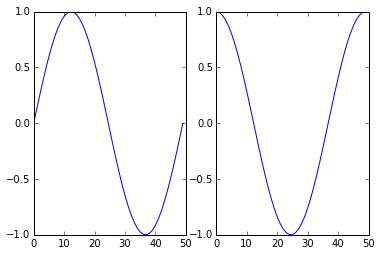## 向图中添加数据¶

In :
plot(x)
plot(y)

Out:
[<matplotlib.lines.Line2D at 0xcbcfd30>]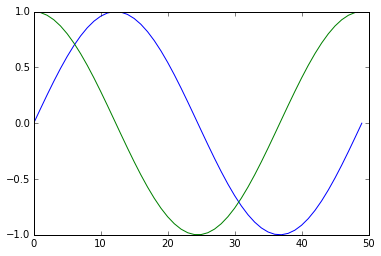In :
plot(x)
hold(False)
plot(y)
# 恢复原来设定
hold(True)

Out:
[<matplotlib.lines.Line2D at 0xcf4b9b0>]## 标签¶

In :
plot(x, label='sin')
plot(y, label='cos')
legend()

Out:
<matplotlib.legend.Legend at 0xd2089b0>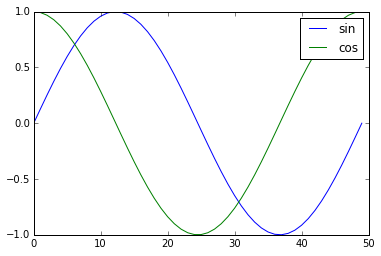In :
plot(x)
plot(y)
legend(['sin', 'cos'])

Out:
<matplotlib.legend.Legend at 0xd51fb00>## 坐标轴，标题，网格¶

In :
plot(x, sin(x))
# 可以设置字体大小
ylabel('amplitude', fontsize='large')
title('Sin(x)')

Out:
<matplotlib.text.Text at 0xd727dd8>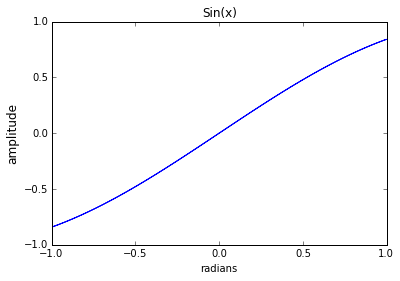In :
plot(x, sin(x))
ylabel('amplitude', fontsize='large')
title('Sin(x)')
grid()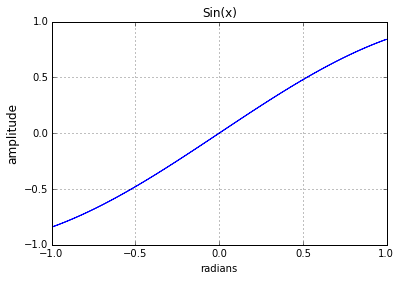## 清除、关闭图像¶

clf()



close()



close('all')

## imshow 显示图片¶

In :
# 导入lena图片
from scipy.misc import lena
img = lena()
img

Out:
array([[162, 162, 162, ..., 170, 155, 128],
[162, 162, 162, ..., 170, 155, 128],
[162, 162, 162, ..., 170, 155, 128],
...,
[ 43,  43,  50, ..., 104, 100,  98],
[ 44,  44,  55, ..., 104, 105, 108],
[ 44,  44,  55, ..., 104, 105, 108]])

In :
imshow(img,
# 设置坐标范围
extent = [-25, 25, -25, 25],
# 设置colormap
cmap = cm.bone)
colorbar()

Out:
<matplotlib.colorbar.Colorbar instance at 0x000000000DECFD88>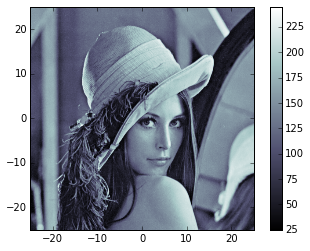In :
dir(cm)

Out:
[u'Accent',
u'Accent_r',
u'Blues',
u'Blues_r',
u'BrBG',
u'BrBG_r',
u'BuGn',
u'BuGn_r',
u'BuPu',
u'BuPu_r',
u'CMRmap',
u'CMRmap_r',
u'Dark2',
u'Dark2_r',
u'GnBu',
u'GnBu_r',
u'Greens',
u'Greens_r',
u'Greys',
u'Greys_r',
'LUTSIZE',
u'OrRd',
u'OrRd_r',
u'Oranges',
u'Oranges_r',
u'PRGn',
u'PRGn_r',
u'Paired',
u'Paired_r',
u'Pastel1',
u'Pastel1_r',
u'Pastel2',
u'Pastel2_r',
u'PiYG',
u'PiYG_r',
u'PuBu',
u'PuBuGn',
u'PuBuGn_r',
u'PuBu_r',
u'PuOr',
u'PuOr_r',
u'PuRd',
u'PuRd_r',
u'Purples',
u'Purples_r',
u'RdBu',
u'RdBu_r',
u'RdGy',
u'RdGy_r',
u'RdPu',
u'RdPu_r',
u'RdYlBu',
u'RdYlBu_r',
u'RdYlGn',
u'RdYlGn_r',
u'Reds',
u'Reds_r',
'ScalarMappable',
u'Set1',
u'Set1_r',
u'Set2',
u'Set2_r',
u'Set3',
u'Set3_r',
u'Spectral',
u'Spectral_r',
u'Wistia',
u'Wistia_r',
u'YlGn',
u'YlGnBu',
u'YlGnBu_r',
u'YlGn_r',
u'YlOrBr',
u'YlOrBr_r',
u'YlOrRd',
u'YlOrRd_r',
'__builtins__',
'__doc__',
'__file__',
'__name__',
'__package__',
'_generate_cmap',
'_reverse_cmap_spec',
'_reverser',
'absolute_import',
u'afmhot',
u'afmhot_r',
u'autumn',
u'autumn_r',
u'binary',
u'binary_r',
u'bone',
u'bone_r',
u'brg',
u'brg_r',
u'bwr',
u'bwr_r',
'cbook',
'cmap_d',
'cmapname',
'colors',
u'cool',
u'cool_r',
u'coolwarm',
u'coolwarm_r',
u'copper',
u'copper_r',
'cubehelix',
u'cubehelix_r',
'division',
u'flag',
u'flag_r',
'get_cmap',
u'gist_earth',
u'gist_earth_r',
u'gist_gray',
u'gist_gray_r',
u'gist_heat',
u'gist_heat_r',
u'gist_ncar',
u'gist_ncar_r',
u'gist_rainbow',
u'gist_rainbow_r',
u'gist_stern',
u'gist_stern_r',
u'gist_yarg',
u'gist_yarg_r',
u'gnuplot',
u'gnuplot2',
u'gnuplot2_r',
u'gnuplot_r',
u'gray',
u'gray_r',
u'hot',
u'hot_r',
u'hsv',
u'hsv_r',
u'jet',
u'jet_r',
'ma',
'mpl',
u'nipy_spectral',
u'nipy_spectral_r',
'np',
u'ocean',
u'ocean_r',
'os',
u'pink',
u'pink_r',
'print_function',
u'prism',
u'prism_r',
u'rainbow',
u'rainbow_r',
'register_cmap',
'revcmap',
u'seismic',
u'seismic_r',
'six',
'spec',
'spec_reversed',
u'spectral',
u'spectral_r',
u'spring',
u'spring_r',
u'summer',
u'summer_r',
u'terrain',
u'terrain_r',
'unicode_literals',
u'winter',
u'winter_r']

In :
imshow(img, cmap=cm.RdGy_r)

Out:
<matplotlib.image.AxesImage at 0xe0883c8>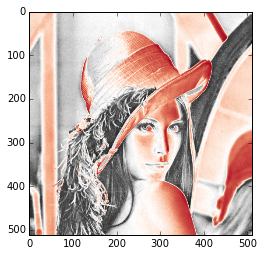## 直方图¶

In :
hist(randn(1000))

Out:
(array([   2.,    7.,   37.,  119.,  216.,  270.,  223.,   82.,   31.,   13.]),
array([-3.65594649, -2.98847032, -2.32099415, -1.65351798, -0.98604181,
-0.31856564,  0.34891053,  1.0163867 ,  1.68386287,  2.35133904,
3.01881521]),
<a list of 10 Patch objects>)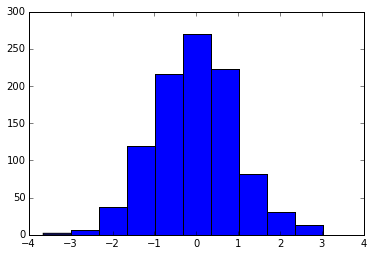http://matplotlib.org/gallery.html